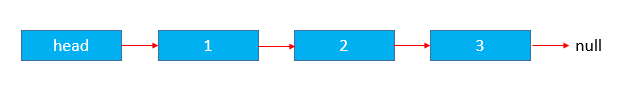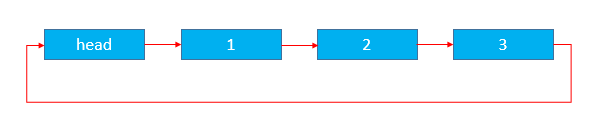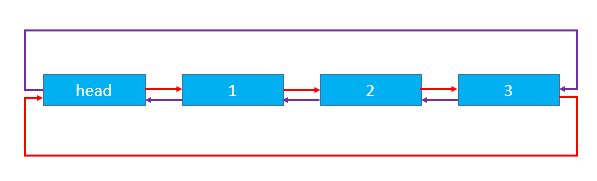## 链表

### 单向链表``  class Node{``      public String data;//链表的数据先采用简单的String``      public Node next;//下一个节点``      public Node(String data){``          this.data = data;``      }``      public void setNext(Node node){``          this.next = node;``      }``      public String getData(){``          return this.data;``      }``      public Node getNext(){``          return this.next;``      }``  }``  public class TestLink{``      public static void main(String[] arg){``          //定义head``          Node head = new Node("我是head");``          Node node1 = new Node("我是node1");``          Node node2 = new Node("我是node2");``          Node node3 = new Node("我是node3");``          head.setNext(node1);//设置head的下一个节点为node1``          node1.setNext(node2);//设置node1的下一个节点为node2``          node2.setNext(node3);//设置node2的下一个节点为node3``          print(head);``      }``   //递归调用打印链表``      public static void print(Node head){``       //当最后一个的getNext()返回null,表示链表已经到底了``          if(head.getNext() == null){``              return;``          }``          System.out.println(head.getData());``          print(head.getNext());``      }``  }``  //java TestLink``  //我是head``  //我是node1``  //我是node2``

### 循环链表``  class Node{``      public String data;//链表的数据先采用简单的String``      public Node next;//下一个节点``      public Node(String data){``          this.data = data;``      }``      public void setNext(Node node){``          this.next = node;``      }``      public String getData(){``          return this.data;``      }``      public Node getNext(){``          return this.next;``      }``  }``  public class TestLink{``      public static void main(String[] arg){``          //定义head``          Node head = new Node("我是head");``          Node node1 = new Node("我是node1");``          Node node2 = new Node("我是node2");``          Node node3 = new Node("我是node3");``          head.setNext(node1);//设置head的下一个节点为node1``          node1.setNext(node2);//设置node1的下一个节点为node2``          node2.setNext(node3);//设置node2的下一个节点为node3``          node3.setNext(head);//设置node3的下一个节点为head 此步完成了循环链表``      }``  }``

### 双向链表``  class Node{``      public String data;//链表的数据先采用简单的String``      public Node pre;//上一个节点``      public Node next;//下一个节点``      public Node(String data){``          this.data = data;``      }``      public void setNext(Node node){``          this.next = node;``      }``      public void setPre(Node node){``          this.pre = node;``      }``      public String getData(){``          return this.data;``      }``      public Node getNext(){``          return this.next;``      }``      public Node getPre(){``          return this.pre;``      }``  }``  public class TestLink{``      public static void main(String[] arg){``          //定义head``          Node head = new Node("我是head");``          Node node1 = new Node("我是node1");``          Node node2 = new Node("我是node2");``          Node node3 = new Node("我是node3");``          head.setNext(node1);//设置head的下一个节点为node1``          node1.setPre(head);//设置node1的上一个节点为head``          node1.setNext(node2);//设置node1的下一个节点为node2``          node2.setPre(node1);//设置node2的上一个节点为node1``          node2.setNext(node3);//设置node1的下一个节点为node2``          node3.setPre(node2);//设置node3即最后一个节点的前一个节点为node2``          node3.setNext(head);//设置node3即最后一个节点的下一个节点为head``          head.setPre(node3);//设置head的上一个节点为node3``      }``  }``